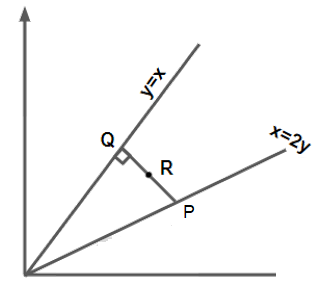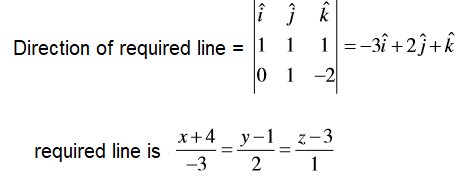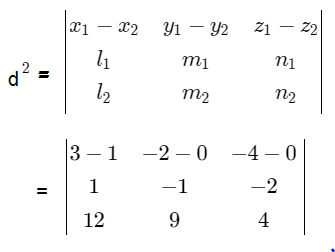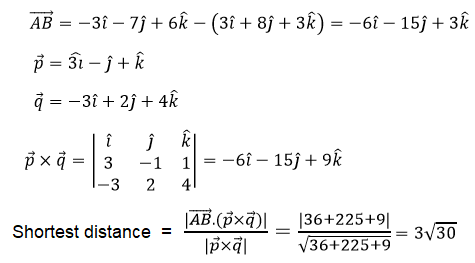# JEE Straight Line Previous Year Questions With Solutions

Straight line is the smallest distance between two points which is not curved in any direction and continuous in the same direction. Straight line is one of the important concepts in JEE syllabus. We at BYJU’S understand the importance well-explained solution modules, hence, we have come up with an ultimate set of previous year question bank on topic straight lines. Straight lines solutions are available for students who are looking to solve questions from JEE past year papers. Students can get step-wise detailed explanation to all the problems in a very simple and easy manner on concepts, perpendicular distance between two lines, point dividing a line segment, normal form of a line, area of triangle, point-slope form of a line, two-point form of a line etc. About 2-3 questions are always asked from this chapter in the JEE exam.

### Points to Remember:

 1. Define straight line? Answer 1: Straight Line is defined as a line with zero curvature. Answer 2: The shortest distance between two points in the same direction is called as a straight line. 2. General Form: Ax + By + C = 0, where, A, B are not equal to zero. 3. Slope: The equation of line with a given slope value m and the y-intercept b is y = mx + b, where m is the slope of the line. 4. Shortest Line Distance: Straight line distance between two points, say A and B having coordinates, (x1, y1) and (x2, y2) is expressed as: AB = $\sqrt{(x_2 – x_1)^2 + (y_2 – y_1)^2}$

## JEE Main Past Year Questions With Solutions on Straight Lines

Questions given below have been asked in the past year JEE entrance exams.

Question 1: If C be the centroid of the triangle having vertices (3,−1), (1,3) and (2, 4). Let P be the point of intersection of the lines x + 3y − 1 = 0 and 3x − y + 1 = 0, then the line passing through the points C and P also passes through the point:

(a) (-9, -7) (b) (-9, -6) (c) (7, 6) (d) (9, 7)

Solution:

Coordinates of C = $(\frac{3+1+2}{3}, \frac{-1+3+4}{3})$ = (2, 2)

Point of intersection of two lines: x + 3y – 1 = 0 and 3x – y + 1 = 0 is A(-1/2, 2/5)

Now, equation of line CA is 8x – 11y + 6 = 0

and Point (-9, -6) lies on CP.

Question 2: The locus of mid points of the perpendiculars drawn from points on the line x = 2y to the line x = y is:

(a) 2x – 3y = 0

(b) 3x − 2y = 0

(c) 5x − 7y = 0

(d) 7x − 5y = 0

Solution:Let R be the midpoint of PQ

PQ is perpendicular on line: y = x

Therefore, the equation of the line PQ can be written as

y = −x + c and it intersects y = x at point Q and x = 2y at point P.

(c/2, c/2) and (2c/3, c/3) are the Coordinates of points Q and P respectively.

Now, Midpoint R = (7c/12, 5c/12) and

Locus of R: x = 7c/12, y = 5c/12

This implies, 5x − 7y = 0

Question 3: Find the equation of line through (-4, 1, 3) and parallel to the plane x + y + z = 3 while the line intersects

another line whose equation is x + y – z = 0 = x + 2y – 3z + 5

(a) $\frac{x+4}{-3} = \frac{y-1}{-2}=\frac{z-3}{1}$

(b) $\frac{x+4}{1} = \frac{y-1}{2}=\frac{z-3}{1}$

(c) $\frac{x+4}{-3} = \frac{y-1}{2}=\frac{z-3}{1}$

(d) $\frac{x+4}{-1} = \frac{y-1}{2}=\frac{z-3}{-3}$

Solution:

Family of planes containing the line of intersection of planes is A + λB = 0

Where A = (x + y – z) and B = (x + 2y – 3z + 5)

That is,

(x + y – z) + λ(x + 2y – 3z + 5) = 0

Above line passing through the point (-4, 1, 3), so satisfy the equation.

On substituting the above equation, we get

λ = -1

=> Equation of plane is y – 2z + 5 = 0

Required line is lie in this plane and is parallel to x + y + z = 5.

Now,Question 4: A plane parallel to y-axis passing through line of intersection of planes x + y + z = 1 and 2x + 3y – z – 4 = 0 which of the point lie on the plane.

(a) (3, 2, 1)                 (b) (-3, 0, 1)                    (c) (-3, 1, 1)                        (d) (3, 1, -1)

Solution:

Required plane is A + λB = 0

Where A = (x + y + z – 1) and B = (2x + 3y – z – 4)

That is,

(x + y + z – 1) + λ(2x + 3y – z – 4) = 0

=> (1 + 2λ)x + (1 + 3λ)y + (1 – λ)z – (1 + 4λ) = 0

As above equation is parallel to y-axis, so λ = -1/3

Therefore, required plane is x + 4z + 1 = 0

Using given all options, we found that (3, 1, -1) is the point lie in this plane.

Question 5: If the foot of perpendicular drawn from the point (1, 0, 3) on a line passing through (α, 7, 1) is (5/3, 7/3, 17/3), then What will be the value of α.

Solution:

Let P(1, 0, 3) and Q(5/3, 7/3, 17/3) are given points.

Direction ratios of line L is:

(α – 5/3, 7 – 7/3, 1 – 17/3) = ( (3α – 5)/3, 14/3, -14/3 )

Direction ratios of PQ: (-2/3, -7/3, -8/3)

Since line L is perpendicular to PQ then

$\frac{3 \alpha -5}{3} \times \frac{-2}{3} + \frac{14}{3} \times \frac{-7}{3} + \frac{-14}{3} \times \frac{-8}{3} = 0$

=> -6α +10 – 98 + 112 = 0

=> 6α = 24

=> α = 4

Therefore, the value of α is 4.

Question 6: If the line, (x-3)/1 = (y-2)/-1 = (z+λ)/-2 lie in the plane, 2x – 4y + 3z = 2, then the shortest distance between this line and the line (x-1)/12 = y/9 = z/4 is:

(a) 2                           (b) 1                             (c) 0                      (d) 3

Solution:

(x-3)/1 = (y-2)/-1 = (z+λ)/-2 = k

Let P be any point on the plane,

P = (k + 3, -k – 2, -2k – λ )

P lie on the plane, 2x – 4y + 3z = 2, Which means P satisfy the equationn of plane.

So, 2(k + 3) – 4(-k – 2) + 3(-2k – λ) = 2

Solving above equation, we have λ = 4

Now, the shortest distance between the below lines:

(x-3)/1 = (y-2)/-1 = (z+4)/-2 and (x-1)/12 = y/9 = z/4 is= |2(14) + 2(28) – 4(21)|

= 0

=> d2 = 0

or d = 0

Question 7: Let A(1, 0), B(6, 2), C(3/2, 6) be the vertices of a triangle ABC. If P is a point inside the triangle ABC such that the triangles APC, APB and BPC have equal areas, then find the length of the line segment PQ, where 𝑄 is the point (-7/6, -1/3).

Solution:

Since point P is the centroid, so its coordinates are

$(\frac{1+6+3/2}{3}, \frac{1+5+2}{3})$ = (17/6, 8/3)

and coordinates of point Q : (-7/6, -1/3)

Now, PQ = √[42+32] = 5

Question 8: Let two points be A(1, −1) and B(0, 2). If a point P(x’, y’) be such that the area of triangle PAB = 5 sq. units and it lies on the line, 3x + y – 4λ = 0, then the value of λ is

(a) 4                       (b) 1                           (c) -3                    (d) 3

Solution:

Let two points be A(1, −1) and B(0, 2). If a point P(x’, y’) be such that the area of triangle PAB = 5 sq. units

Area of triangle is:=> (2 – y’) – x’ – 2x’ = ± 10

=> 3x’ + y’ = 12 or 3x’ + y’ = -8

Since triangle lie on the line 3x + y – 4λ = 0 then,

=> λ = 3 or λ = -2

Neglecting negative value.

So, λ = 3

Question 9: Find the shortest distance between the lines:

$\frac{x-3}{3}=\frac{y-8}{-1}=\frac{z-3}{1}$ and $\frac{x+3}{-3}=\frac{y+7}{2}=\frac{z-6}{4}$

Solution:Question 10: The length of the perpendicular from the origin, on the normal to the curve, x2 + 2xy – 3y2 = 0 at the point (2,2) is:

(a) 2                       (b) 2√2                    (c) 4√2                      (d) √2

Solution:

Given curve: x2 + 2xy – 3y2 = 0

=> x2 + 3xy – xy – 3y2 = 0

=> x(x + 3y) – y(x + 3y) = 0

=> (x + 3y)(x – y) = 0

=> x + 3y = 0 or x – y = 0

Since point (2, 2) lies on x – y = 0

Therefore,

Equation of normal will be x + y = λ

Put x = 2 and y = 2

So, λ = 4

Required line is x + y = 4

Now, Distance of L from the origin = |−4/√2 | = 2√2